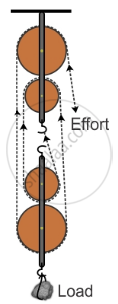Share
Notifications

View all notifications
Books Shortlist
Your shortlist is empty

# A Block and Tackle System Has the Velocity Ratio 3. a Man Can Exert a Pull of 200 Kgf. What is the Maximum Load He Can Raise with this Pulley System is Its Efficiency is 60%? - ICSE Class 10 - Physics

Login
Create free account

Forgot password?

#### Question

A block and tackle system has the velocity ratio 3. Draw a labelled diagram of the system indicating the points of application and the directions of load and effort. A man can exert a pull of 200 kgf. What is the maximum load he can raise with this pulley system is its efficiency is 60%?

#### SolutionA block and tackle system has the velocity ratio = 3
i.e., VR = n = 3

Efficiency of system η = 60% = 0.6
The mechanical advantage of the system MA. = V.A × η = 3 × 0.6 = 1.8
Man can exert a maximum effort = 200 kgf
Load = M.A. × effort = 1.8 × 200 = 360 kgf

Is there an error in this question or solution?

#### APPEARS IN

Solution A Block and Tackle System Has the Velocity Ratio 3. a Man Can Exert a Pull of 200 Kgf. What is the Maximum Load He Can Raise with this Pulley System is Its Efficiency is 60%? Concept: Concept of Pulley System.
S